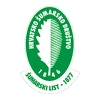DIGITALNA ARHIVA ŠUMARSKOG LISTA
prilagođeno pretraživanje po punom tekstu

 ŠUMARSKI LIST 7-8/2015 str. 54     <-- 54 -->        PDF characteristics. Additionally, by using the productivity data we compared the efficiency of both technologies, separated by the general stand characteristics determined by morphological characters of the trees. In the mechanized cutting operation we recorded the share of 62.9 % of productive time, out of which the Main work time (Felling and processing) was 64.7 % while the rest pertained to Complementary work time 35.3 % (Driving in the stand /site, Positioning of the machine, Positioning of the harvester head). The structure of Work Place time with respect to Work time and Non-work time is within limits for similar operations elsewhere. The impact of individual factors which are related to tree characteristics was studied by the analysis of the Main Work time (Felling and processing). The analysis of felling and processing time has included the following independent variables: DBH, crown length, branch thickness and stem form. The distribution of cut trees by influential factor classes is shown in Table 6. By regression analysis we determined which of the influential factors has a statistically significant impact on felling and processing. The results showed that the linear regression model is significant for explanation of efficiency in Main Work Time. The model explains 76 % variance (R2 = 0.76), but the contributions of individual variables are very different (Table 7). The variables with a statistically significant impact are the breast height diameter (DBH) and stem form. We conducted the analysis of covariance where the dependent variable was felling and processing time. We used DBH as covariate; the stem form was used as an independent variable which also has statistically significant influence on productivity. Furthermore, we tested the level of interaction between independent variable (stem form) and covariate (DBH). The analysis of the characteristics of the interaction between covariate (DBH) and independent variable (stem form) shows the atypical interaction of both variables. Even partial Eta squared is close to zero and this may further confirm the assumption that there is a small proportion of variation explained by the interaction of both variables. Therefore, we can accept the assumption that the covariate variable (DBH) is homogeneously distributed within the value of independent variable (steam form). In Table 8 the average values of Main productive time by stem form classes for CTL technology are shown. The expectation of increasing average main productive time consumption by the classes of stem forms are confirmed – that is by a growing class of tree forking. The statistical tests were conducted in order to qualify the conditions of the performance in the covariance analysis (Table 9). The analysis of covariance shows that the stem form has a statistically significant impact on work element Felling and